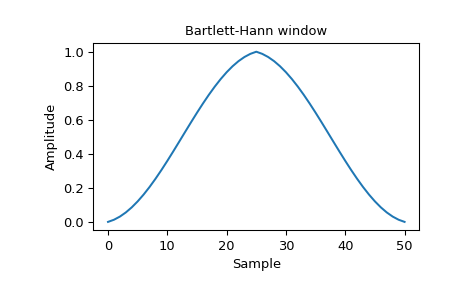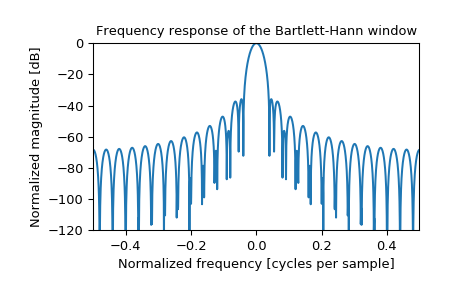# scipy.signal.windows.barthann¶

scipy.signal.windows.barthann(M, sym=True)[source]

Return a modified Bartlett-Hann window.

Parameters
Mint

Number of points in the output window. If zero or less, an empty array is returned.

symbool, optional

When True (default), generates a symmetric window, for use in filter design. When False, generates a periodic window, for use in spectral analysis.

Returns
wndarray

The window, with the maximum value normalized to 1 (though the value 1 does not appear if M is even and sym is True).

Examples

Plot the window and its frequency response:

>>> from scipy import signal
>>> from scipy.fftpack import fft, fftshift
>>> import matplotlib.pyplot as plt

>>> window = signal.barthann(51)
>>> plt.plot(window)
>>> plt.title("Bartlett-Hann window")
>>> plt.ylabel("Amplitude")
>>> plt.xlabel("Sample")

>>> plt.figure()
>>> A = fft(window, 2048) / (len(window)/2.0)
>>> freq = np.linspace(-0.5, 0.5, len(A))
>>> response = 20 * np.log10(np.abs(fftshift(A / abs(A).max())))
>>> plt.plot(freq, response)
>>> plt.axis([-0.5, 0.5, -120, 0])
>>> plt.title("Frequency response of the Bartlett-Hann window")
>>> plt.ylabel("Normalized magnitude [dB]")
>>> plt.xlabel("Normalized frequency [cycles per sample]")